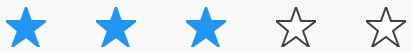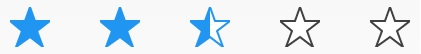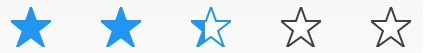# Precision Mode in Xamarin Rating (SfRating)

4 Aug 20221 minute to read

The `Precision` mode defines the accuracy level of the `SfRating` control. It has `Standard`, `Half`, and `Exact` options. The default precision mode of the `SfRating` control is `Standard`.

## Standard

When the precision mode of `SfRating` is set as `Standard`, the rating item will be filled completely based on the rating value.

``<rating:SfRating x:Name="rating" Precision="Standard" />``
``````SfRating rating;
public MainPage()
{
InitializeComponent();
rating = new SfRating();
rating.Precision = Precision.Standard;
}``````## Half

When the precision mode of `SfRating` is set as `Half`, the rating item will be filled partially based on the rating value.

``<rating:SfRating x:Name="rating" Precision="Half" />``
``````SfRating rating;
public MainPage()
{
InitializeComponent();
rating = new SfRating();
rating.Precision = Precision.Half;
}``````## Exact

When the precision mode of `SfRating` is set as `Exact`, the rating item will be filled exactly based on the rating value.

``<rating:SfRating x:Name="rating" Precision="Exact" />``
``````SfRating rating;
public MainPage()
{
InitializeComponent();
rating = new SfRating();
rating.Precision = Precision.Exact;
}``````